# NCERT Solutions class 7 Maths Chapter-13 Exercise 13.2

## NCERT Solutions class 7 Maths Chapter-13 Exponents and Powers

### Exponents and Powers Exercise-13.2

NCERT Solutions Class-7 Maths chapter-13 Exponents and Powers Exercise-13.2 is prepared by academic team of pw all the questions of NCERT text book are solved step by step with proper and detail solutions explaining each and every questions . For More and additional questions of CBSE class 7 maths you can go to class 7 maths sections. NCERT class 7 Maths Solutions is the best way to enhanced your mathematics skill. And pw practice worksheet & question bank will help you a lot .

### Solutions of Chapter  Exponents and Powers  Exercise-13.2

Question 1:

Using laws of exponents simplify and write the answer in exponential form:

(i) 32 × 34 × 38

(ii)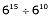(iii)  a× a2

(iv) 7x × 72

(v)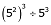(vi)25 × 52

(vii)a4 × b4

(viii)(34)3

(ix)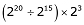(x)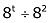(i) We have,

32× 34 × 38

We know that,

(am× an = am + n)

Thus,

32× 34 × 38

= (3)2 + 4 + 8

= 314

(ii) We have,

615 610

We know that,

(am an = am - n)

Thus,

615 610

= (6)15 - 10

= 65

(iii) We have,

a3× a2

We know that,

(am× an = am + n)

Therefore,

a3× a2

= (a)3 + 2

= a5

(iv) We have,

7x × 72

We know that,

(am × an = am + n)

Thus,

7x × 72

= (7)x + 2

(v) We have,

(52)3 53

Using identity:

(am)n= am × n

= 52 × 3 53

= 56 53

We know that,

(am an = am - n)

Thus,

56 53

= (5)6 - 3

= 53

(vi) We have,

25× 55

We know that,

[am ×bm = (a × b)m]

Thus,

25× 55

= (2 × 5)5 + 5

= 105

(vii) We have,

a4 × b4

We know that,

[am × bm = (a × b)m]

Thus,

a4 × b4

= (a × b)4

(viii) We have,

(34)3

We know that,

(am)n = amn)

Thus,

(34)3

= (34)3

= 312

(ix) We have,

(220 215) × 23

We know that,

(am an = am - n)

Thus,

(220 - 15) × 23

= (2)5 × 23

We know that,

(am × an = am + n)

Thus,

(2)5 × 23

=(25 + 3)

= 28

(x) We have,

(8t 82)

We know that,

(am an = am - n)

Thus,

(8t 82)

= (8t – 2)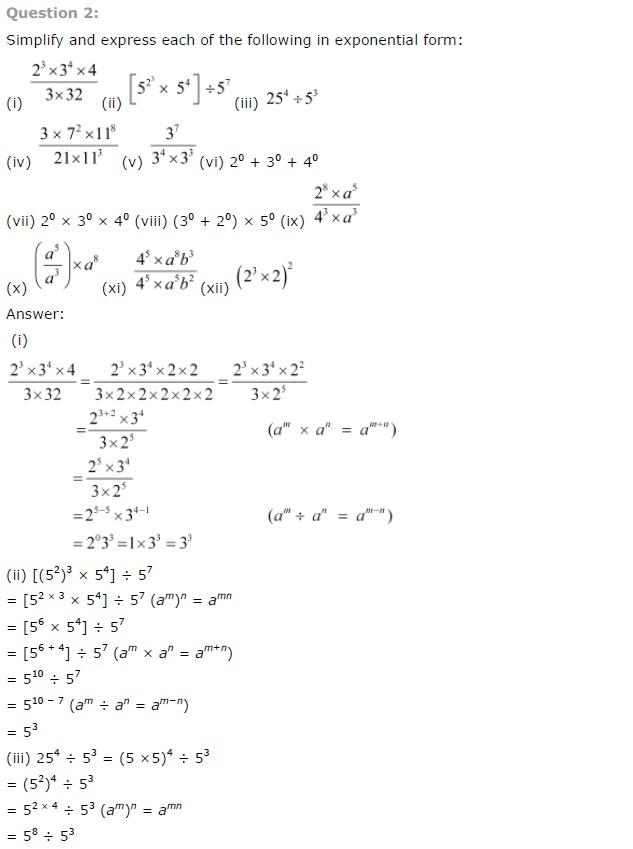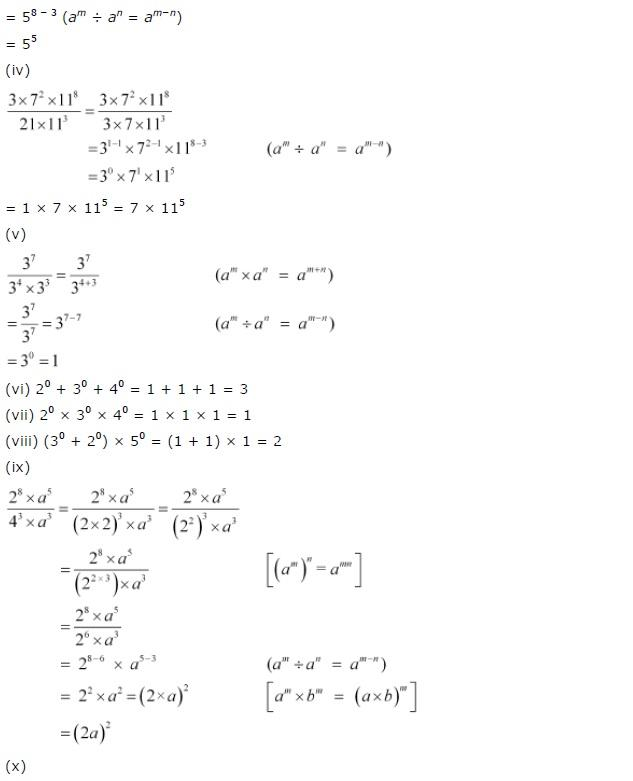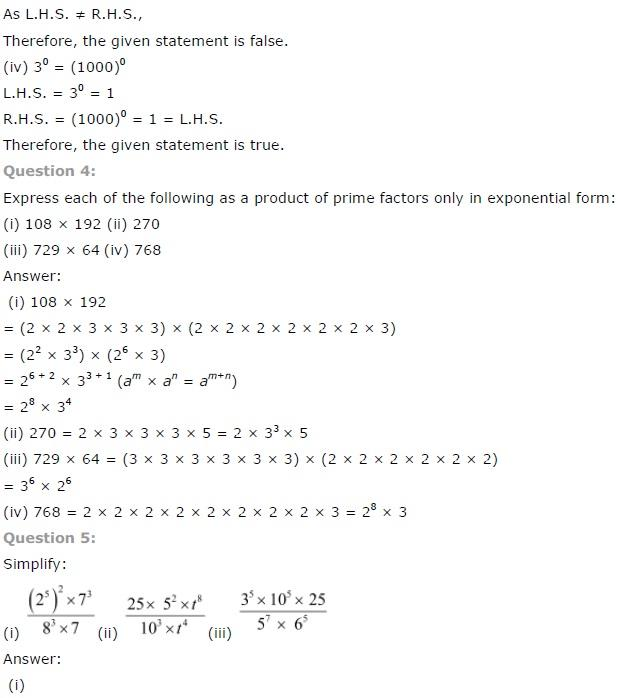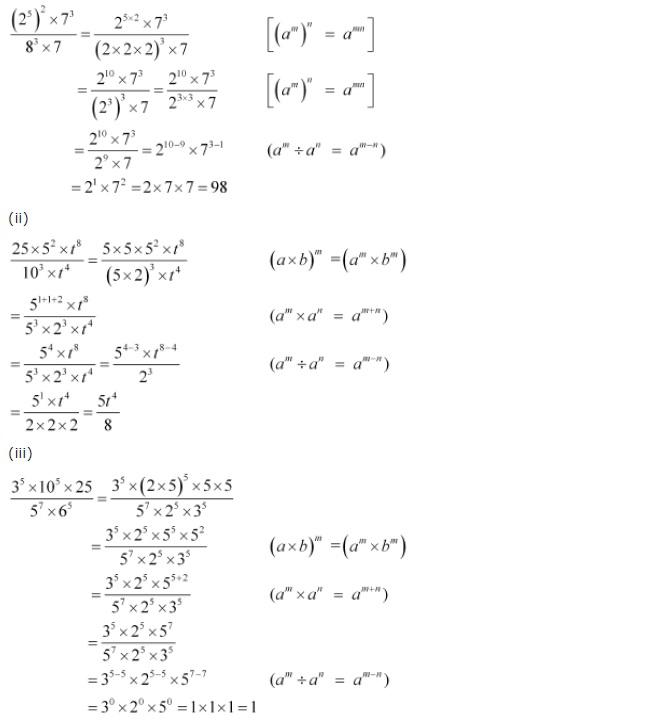NCERT CLASS 7 MATHEMATICS SOLUTIONS